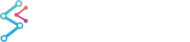﻿ Axis Types in SciChart.js | JavaScript Chart DocumentationSciChart.js JavaScript 2D Charts API > Axis APIs > Axis Types in SciChart.js
Axis Types in SciChart.js

There are several axis types in SciChart.js. Although they all differ in types of data values that can be rendered, the most fundamental difference is in their behavior. By that, the axes can be divided into two groups, Category and Value axis types. Please read the Value Axis vs. Category Axis article elaborating on what the difference is.

The axis types provided by SciChart Android are listed below:

 Axis Type Value or Category Axis NumericAxis Value Axis / Numeric Types DateTimeNumericAxis Value Axis with additional features for Dates and Times CategoryAxis Category Axis / Numeric Types or Dates LogarithmicAxis Logarithmic / Numeric Axis

All the axis types in SciChart inherit from AxisCore - a common type shared across both 2D and 3D Charts - and all 2D Axis inherit AxisBase2D.

## NumericAxis

The NumericAxis is a Value axis and is suitable for X and Y Axis when the data on that axis is numeric (e.g. number in TypeScript). It is not suitable for non-numeric data types.

To create and configure a NumericAxis, use the following code:

NumericAxis
Copy Code
```import {SciChartSurface} from "scichart/Charting/Visuals/SciChartSurface";
import {NumericAxis} from "scichart/Charting/Visuals/Axis/NumericAxis";
import {EAutoRange} from "scichart/types/AutoRange";
import {EAxisAlignment} from "scichart/types/AxisAlignment";
export async function initNumericAxis() {
const { sciChartSurface, wasmContext } = await SciChartSurface.create("scichart-div-1");
// Creating a NumericAxis as an XAxis on the bottom
drawMajorGridLines: true,
drawMinorGridLines: true,
axisTitle: "X Axis",
axisAlignment: EAxisAlignment.Bottom,
autoRange: EAutoRange.Once,
}));
// Creating a NumericAxis as a YAxis on the left
drawMajorGridLines: true,
drawMinorGridLines: true,
axisTitle: "Y Axis",
axisAlignment: EAxisAlignment.Left,
autoRange: EAutoRange.Once,
}));
}
```

## NumericAxis - with Date Formatting

A NumericAxis can also be used to format dates. To do this, the data in your DataSeries must be a number in Unix date format, then the number may be formatted as date using the LabelProvider feature.

NumericAxis
Copy Code
```import {SciChartSurface} from "scichart/Charting/Visuals/SciChartSurface";
import {NumericAxis} from "scichart/Charting/Visuals/Axis/NumericAxis";
import {EAutoRange} from "scichart/types/AutoRange";
import {EAxisAlignment} from "scichart/types/AxisAlignment";
import {NumericLabelProvider} from "scichart/Charting/Visuals/Axis/LabelProvider/NumericLabelProvider";
import {ENumericFormat} from "scichart/types/NumericFormat";
export async function initNumericAxisWithDates() {
const { sciChartSurface, wasmContext } = await SciChartSurface.create("scichart-div-2");
// Create an XAxis on the bottom
const xAxis = new NumericAxis(wasmContext, {
drawMajorGridLines: true,
drawMinorGridLines: true,
axisTitle: "X Axis",
axisAlignment: EAxisAlignment.Bottom,
autoRange: EAutoRange.Once,
});
// Alternative Api. Set a LabelProvider with date formatting
// see the labelprovider docs for more advanced API
xAxis.labelProvider = new NumericLabelProvider({
labelFormat: ENumericFormat.Date_DDMMYY,
cursorLabelFormat: ENumericFormat.Date_DDMMYYYY,
});
// Add the xAxis to the chart
// Creating a NumericAxis as a YAxis on the left
axisTitle: "Y Axis",
axisAlignment: EAxisAlignment.Left,
}));
}
```

A subclass of NumericAxis is the DateTimeNumericAxis - this can also be used to format dates. To do this, the data in your DataSeries must be a number in Unix date format, then the number may be formatted as date using the LabelProvider feature.

NumericAxis
Copy Code
```import {SciChartSurface} from "scichart/Charting/Visuals/SciChartSurface";
import {EAutoRange} from "scichart/types/AutoRange";
import {EAxisAlignment} from "scichart/types/AxisAlignment";
import {NumericLabelProvider} from "scichart/Charting/Visuals/Axis/LabelProvider/NumericLabelProvider";
import {ENumericFormat} from "scichart/types/NumericFormat";
export async function initNumericAxisWithDates() {
const { sciChartSurface, wasmContext } = await SciChartSurface.create("scichart-div-2");
// Create an XAxis on the bottom
const xAxis = new DateTimeNumericAxis(wasmContext, {
drawMajorGridLines: true,
drawMinorGridLines: true,
axisTitle: "X Axis",
axisAlignment: EAxisAlignment.Bottom,
autoRange: EAutoRange.Once,
});
// Add the xAxis to the chart
// Creating a NumericAxis as a YAxis on the left
axisTitle: "Y Axis",
axisAlignment: EAxisAlignment.Left,
}));
}
```

## CategoryAxis

The CategoryAxis is a Category axis and is suitable for the XAxis only. It is designed to handle a special case when data is discontinuous or contains breaks at regular intervals. Unlike the other axis types, it works with with data indexes, not actual data values. It is not suitable for YAxis.

## LogarithmicAxis

The LogarithmicAxis is a special numeric axis type which displays values in Logarithmic Scale. To create and configure a CategoryAxis, use the following code:

LogarithmicAxis
Copy Code
```import {SciChartSurface} from "scichart/Charting/Visuals/SciChartSurface";
import {NumericAxis} from "scichart/Charting/Visuals/Axis/NumericAxis";
import {EAxisAlignment} from "scichart/types/AxisAlignment";
import {ENumericFormat} from "scichart/types/NumericFormat";
import {LogarithmicAxis} from "scichart/Charting/Visuals/Axis/LogarithmicAxis";
import {NumberRange} from "scichart/Core/NumberRange";
export async function initLogAxis() {
const { sciChartSurface, wasmContext } = await SciChartSurface.create("scichart-div-4");
// Creating a Log(10) Axis for xAxis
axisTitle: "Log(10) Axis",
axisAlignment: EAxisAlignment.Bottom,
labelFormat: ENumericFormat.Scientific,
logBase: 10,
visibleRange: new NumberRange(1, 1E6)
}));
// Creating a Log(2) Axis on the YAxis
axisTitle: "Log(2) Axis",
axisAlignment: EAxisAlignment.Left,
labelFormat: ENumericFormat.Exponential,
logBase: 2,
visibleRange: new NumberRange(1, 512)
}));
}
```

The LogarithmicAxis has it's own documentation page to go over all the options. For more info see here.

## Multiline Axis Titles

SciChart.JS v2 allows multiline axis titles, by simply passing an array of lines as the axis title.

Multiline Axis title
Copy Code
```xAxis.axisTitle = ["This is a very long axis title", "that has been split into multiple lines", "to demonstrate the new functionality"];
// Axis titles are 24px by default.  Multi-line ones may need to be smaller
xAxis.axisTitleStyle.fontSize = 16;
```

## See the Axis Types in Action

Please take a look at the examples from the SciChart.js Examples Suite listed below to see these axis types in action: